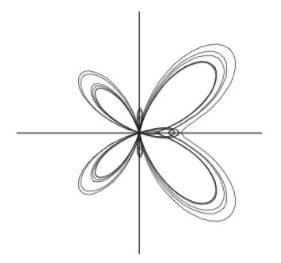Chapter 10, Problem 16PS

Chapter
Section
Textbook Problem

# Butterfly Curve Use a graphing utility to graph the curve shown in the figure below. The curve is given by r = e cos θ − 2 cos 4 θ + sin 5 θ 12 . Over what interval must θ vary to produce the curve?To determine

To graph: The polar curve r=ecosθ2cos4θ+sin5θ12 and determine the range of θ where the curve is identical to the one provided.

Explanation

Given: The graph:

Graph:

Use the Ti-83 calculator to sketch the graph:

Step 1: Press the [MODE] key and the select the Polar curve option.

Step 2: Press the [Y=] key and then enter the provided equation to the right of Y1.

Step 3: Press [WINDOW] and enter the following values

### Still sussing out bartleby?

Check out a sample textbook solution.

See a sample solution

#### The Solution to Your Study Problems

Bartleby provides explanations to thousands of textbook problems written by our experts, many with advanced degrees!

Get Started

#### Evaluate the integral. 01(xe+ex)dx

Single Variable Calculus: Early Transcendentals, Volume I

#### Solve the equations in Exercises 126. x4x+1xx1=0

Finite Mathematics and Applied Calculus (MindTap Course List)

#### What is the lowest score in the following distribution?

Essentials of Statistics for The Behavioral Sciences (MindTap Course List)

#### In problems 23-26, find. 26.

Mathematical Applications for the Management, Life, and Social Sciences

#### Find the limit of the sequence {2,22,222,}

Single Variable Calculus: Early Transcendentals